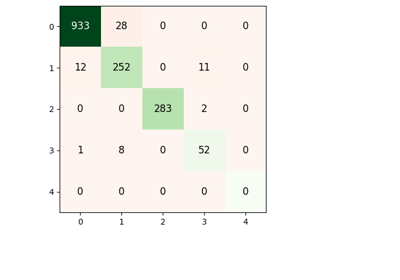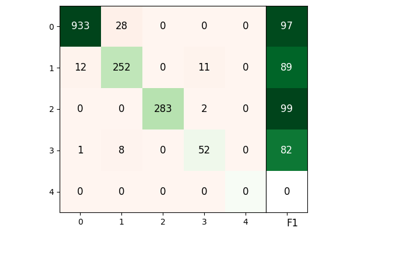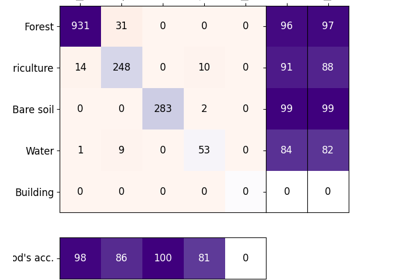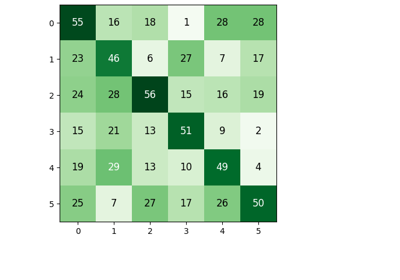# PlotConfusionMatrix¶

class `museotoolbox.charts.``PlotConfusionMatrix`(cm, cmap=<matplotlib.colors.LinearSegmentedColormap object>, left=None, right=None, **kwargs)[source]

Plot a confusion matrix with imshow of pyplot. Customize color (e.g. diagonal color), add subplots with F1 or Producer/User accuracy.

Examples

```>>> plot = mtb.charts.plotConfusionMatrix([[5,6],[1,8]])
 `__init__`(cm[, cmap, left, right]) Initialize self. `add_accuracy`([thresold]) Add user and producer accuracy. Add F1 subplot. `add_mean`([xLabel, yLabel, hide_ticks, …]) Add Mean for both axis. `add_text`([thresold, font_size, alpha, …]) Add value of each case on the matrix image. `add_x_labels`([labels, rotation, position]) Add labels for X. `add_y_labels`([labels, rotation]) Add labels for Y. `color_diagonal`([diag_color, matrix_color]) Add user and producer accuracy. `save_to`(path[, dpi]) Save the plot
## Examples using `museotoolbox.charts.PlotConfusionMatrix`¶